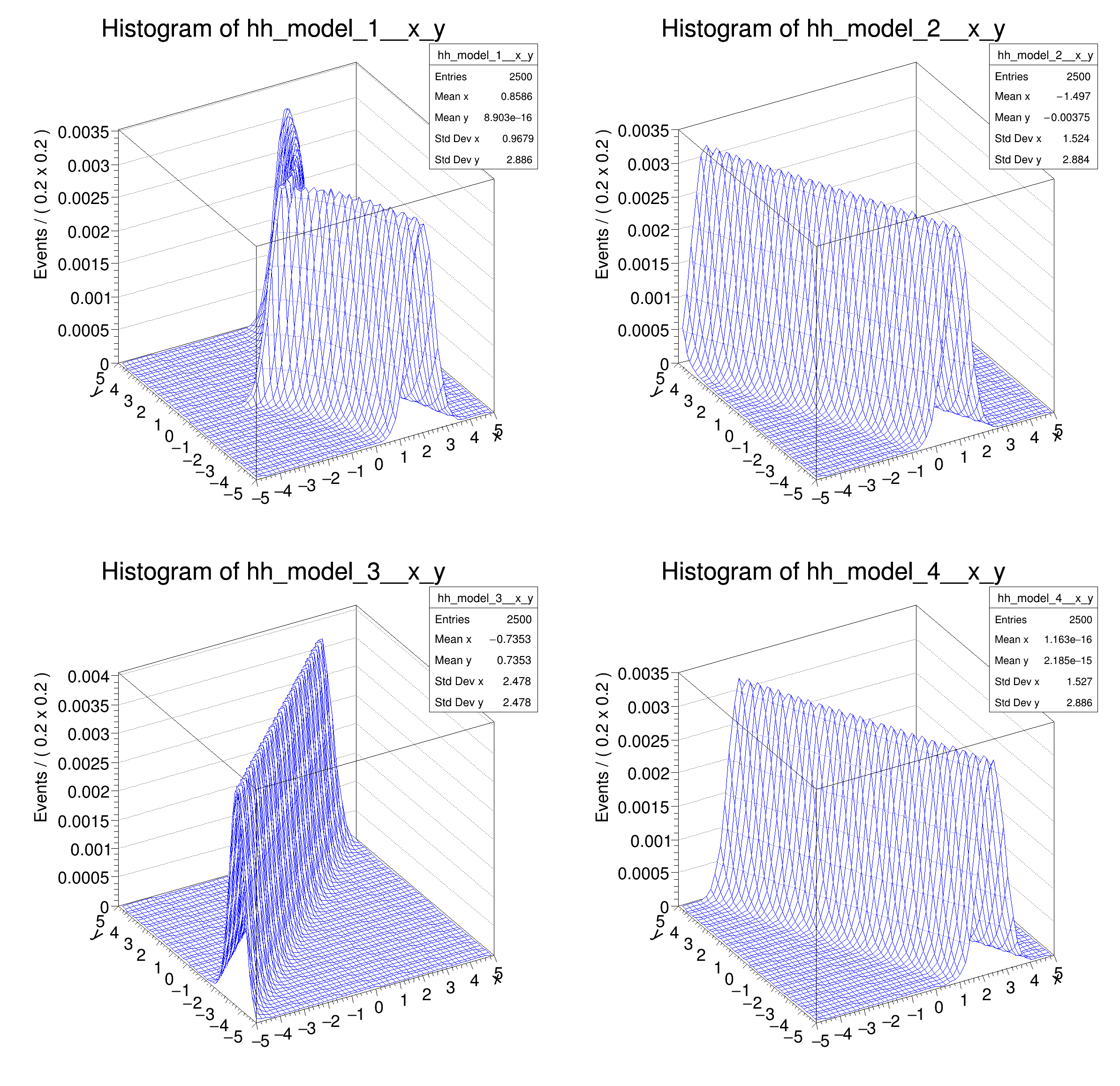ROOT   Reference Guiderf302_utilfuncs.C File Reference

## Detailed DescriptionMultidimensional models: utility functions classes available for use in tailoring of composite (multidimensional) pdfs␛[1mRooFit v3.60 -- Developed by Wouter Verkerke and David Kirkby␛[0m
Copyright (C) 2000-2013 NIKHEF, University of California & Stanford University
[#0] WARNING:InputArguments -- The parameter 'sigma' with range [-1e+30, 1e+30] of the RooGaussian 'model_1' exceeds the safe range of (0, inf). Advise to limit its range.
[#0] WARNING:InputArguments -- The parameter 'sigma' with range [-1e+30, 1e+30] of the RooGaussian 'model_2' exceeds the safe range of (0, inf). Advise to limit its range.
[#0] WARNING:InputArguments -- The parameter 'sigma' with range [-1e+30, 1e+30] of the RooGaussian 'model_3' exceeds the safe range of (0, inf). Advise to limit its range.
[#0] WARNING:InputArguments -- The parameter 'sigma' with range [-1e+30, 1e+30] of the RooGaussian 'model_4' exceeds the safe range of (0, inf). Advise to limit its range.
[#1] INFO:NumericIntegration -- RooRealIntegral::init(model_1_Int[x,y]) using numeric integrator RooIntegrator1D to calculate Int(y)
[#1] INFO:NumericIntegration -- RooRealIntegral::init(model_2_Int[x,y]) using numeric integrator RooIntegrator1D to calculate Int(y)
[#1] INFO:NumericIntegration -- RooRealIntegral::init(model_3_Int[x,y]) using numeric integrator RooIntegrator1D to calculate Int(y)
[#1] INFO:NumericIntegration -- RooRealIntegral::init(model_4_Int[x,y]) using numeric integrator RooIntegrator1D to calculate Int(y)
#include "RooRealVar.h"
#include "RooDataSet.h"
#include "RooGaussian.h"
#include "TCanvas.h"
#include "TAxis.h"
#include "RooPlot.h"
#include "RooFormulaVar.h"
#include "RooProduct.h"
#include "RooPolyVar.h"
#include "TCanvas.h"
#include "TAxis.h"
#include "TH1.h"
using namespace RooFit;
{
// C r e a t e o b s e r v a b l e s , p a r a m e t e r s
// -----------------------------------------------------------
// Create observables
RooRealVar x("x", "x", -5, 5);
RooRealVar y("y", "y", -5, 5);
// Create parameters
RooRealVar a0("a0", "a0", -1.5, -5, 5);
RooRealVar a1("a1", "a1", -0.5, -1, 1);
RooRealVar sigma("sigma", "width of gaussian", 0.5);
// U s i n g R o o F o r m u l a V a r t o t a i l o r p d f
// -----------------------------------------------------------------------
// Create interpreted function f(y) = a0 - a1*sqrt(10*abs(y))
RooFormulaVar fy_1("fy_1", "a0-a1*sqrt(10*abs(y))", RooArgSet(y, a0, a1));
// Create gauss(x,f(y),s)
RooGaussian model_1("model_1", "Gaussian with shifting mean", x, fy_1, sigma);
// U s i n g R o o P o l y V a r t o t a i l o r p d f
// -----------------------------------------------------------------------
// Create polynomial function f(y) = a0 + a1*y
RooPolyVar fy_2("fy_2", "fy_2", y, RooArgSet(a0, a1));
// Create gauss(x,f(y),s)
RooGaussian model_2("model_2", "Gaussian with shifting mean", x, fy_2, sigma);
// U s i n g R o o A d d i t i o n t o t a i l o r p d f
// -----------------------------------------------------------------------
// Create sum function f(y) = a0 + y
// Create gauss(x,f(y),s)
RooGaussian model_3("model_3", "Gaussian with shifting mean", x, fy_3, sigma);
// U s i n g R o o P r o d u c t t o t a i l o r p d f
// -----------------------------------------------------------------------
// Create product function f(y) = a1*y
RooProduct fy_4("fy_4", "a1*y", RooArgSet(a1, y));
// Create gauss(x,f(y),s)
RooGaussian model_4("model_4", "Gaussian with shifting mean", x, fy_4, sigma);
// P l o t a l l p d f s
// ----------------------------
// Make two-dimensional plots in x vs y
TH1 *hh_model_1 = model_1.createHistogram("hh_model_1", x, Binning(50), YVar(y, Binning(50)));
TH1 *hh_model_2 = model_2.createHistogram("hh_model_2", x, Binning(50), YVar(y, Binning(50)));
TH1 *hh_model_3 = model_3.createHistogram("hh_model_3", x, Binning(50), YVar(y, Binning(50)));
TH1 *hh_model_4 = model_4.createHistogram("hh_model_4", x, Binning(50), YVar(y, Binning(50)));
hh_model_1->SetLineColor(kBlue);
hh_model_2->SetLineColor(kBlue);
hh_model_3->SetLineColor(kBlue);
hh_model_4->SetLineColor(kBlue);
// Make canvas and draw RooPlots
TCanvas *c = new TCanvas("rf302_utilfuncs", "rf302_utilfuncs", 800, 800);
c->Divide(2, 2);
c->cd(1);
hh_model_1->GetZaxis()->SetTitleOffset(2.5);
hh_model_1->Draw("surf");
c->cd(2);
hh_model_2->GetZaxis()->SetTitleOffset(2.5);
hh_model_2->Draw("surf");
c->cd(3);
hh_model_3->GetZaxis()->SetTitleOffset(2.5);
hh_model_3->Draw("surf");
c->cd(4);
hh_model_4->GetZaxis()->SetTitleOffset(2.5);
hh_model_4->Draw("surf");
}
#define c(i)
Definition: RSha256.hxx:101
@ kBlue
Definition: Rtypes.h:66
RooAddition calculates the sum of a set of RooAbsReal terms, or when constructed with two sets,...
RooArgSet is a container object that can hold multiple RooAbsArg objects.
Definition: RooArgSet.h:29
A RooFormulaVar is a generic implementation of a real-valued object, which takes a RooArgList of serv...
Definition: RooFormulaVar.h:30
Plain Gaussian p.d.f.
Definition: RooGaussian.h:24
Class RooPolyVar is a RooAbsReal implementing a polynomial in terms of a list of RooAbsReal coefficie...
Definition: RooPolyVar.h:28
A RooProduct represents the product of a given set of RooAbsReal objects.
Definition: RooProduct.h:29
RooRealVar represents a variable that can be changed from the outside.
Definition: RooRealVar.h:39
virtual void SetTitleOffset(Float_t offset=1)
Set distance between the axis and the axis title.
Definition: TAttAxis.cxx:293
virtual void SetLineColor(Color_t lcolor)
Set the line color.
Definition: TAttLine.h:40
The Canvas class.
Definition: TCanvas.h:23
TH1 is the base class of all histogram classes in ROOT.
Definition: TH1.h:58
TAxis * GetZaxis()
Definition: TH1.h:322
virtual void Draw(Option_t *option="")
Draw this histogram with options.
Definition: TH1.cxx:3073
RooCmdArg YVar(const RooAbsRealLValue &var, const RooCmdArg &arg=RooCmdArg::none())
RooCmdArg Binning(const RooAbsBinning &binning)
const Double_t sigma
Double_t y[n]
Definition: legend1.C:17
Double_t x[n]
Definition: legend1.C:17
The namespace RooFit contains mostly switches that change the behaviour of functions of PDFs (or othe...
Date
July 2008

Definition in file rf302_utilfuncs.C.